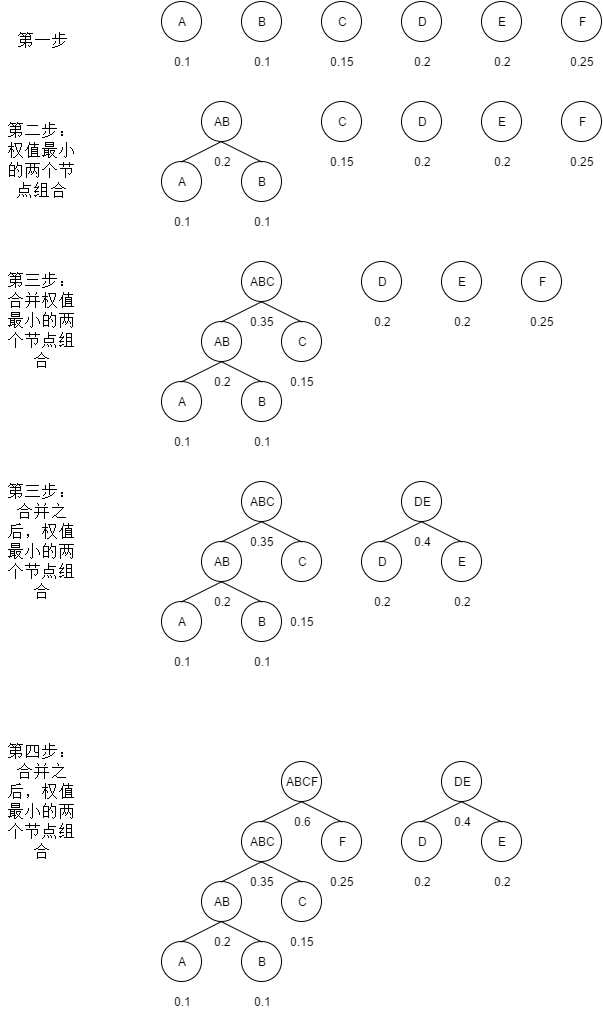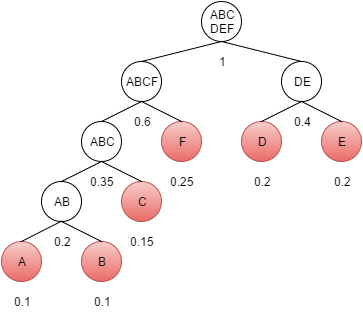# 【H.264/AVC视频编解码技术详解】七、 熵编码算法（1）：基础知识

## 1. 熵编码概念

“熵”这一概念原本来自于化学和热力学，用于度量能量退化的指标，即熵越高，物体或系统的做功能力越低。后来香农将这一概念引入到信息论中，用于表示消息的平均信息量。信源的熵通常可以表示信源所发出信息的不确定性，即越是随机的、前后不相关的信息，其熵越高。

## 2. 熵编码的简单实现——哈夫曼编码

A 0.1
B 0.1
C 0.15
D 0.2
E 0.2
F 0.25• A: 0000
• B: 0001
• C: 001
• D: 10
• E: 11
• F: 01

## 3. 哈夫曼树的构建Demo

#include "stdafx.h"
#include <iostream>
#include <fstream>
#include <queue>
#include <vector>
#include <string>

using namespace std;

//每一个符号定义为一个结构体，包括字符和出现频次
typedef struct
{
unsigned char	character;
unsigned int	frequency;
} CharNode;

static bool open_input_file(ifstream &input, const char *inputFileName)
{
input.open(inputFileName);
if (!input.is_open())
{
return false;
}
return true;
}

struct MinHeapNode
{
char data;
unsigned int freq;
MinHeapNode *left, *right;
MinHeapNode(char data, unsigned freq)
{
left = right = NULL;
this->data = data;
this->freq = freq;
}
};
typedef struct MinHeapNode MinHeapNode;

struct compare
{
bool operator()(MinHeapNode* l, MinHeapNode *r)
{
return (l->freq > r->freq);
}
};

static void get_huffman_code(MinHeapNode *root, string code)
{
if (!root)
{
return;
}

if (root->data != -1)
{
cout << root->data << " : " << code << endl;;
}

get_huffman_code(root->left, code + "0");
get_huffman_code(root->right, code + "1");
}

int _tmain(int argc, _TCHAR* argv[])
{
ifstream inputFile;
if (!open_input_file(inputFile, "input.txt"))
{
cout << "Error: opening input file failed!" << endl;
return -1;
}

char buf = inputFile.get();
CharNode nodeArr = { { 0, 0 } };
while (inputFile.good())
{
cout << buf;
nodeArr[buf].character = buf;
nodeArr[buf].frequency++;
buf = inputFile.get();
}
cout << endl;

priority_queue<MinHeapNode*, vector<MinHeapNode*>, compare>  minHeap;
for (int idx = 0; idx < 256; idx++)
{
if (nodeArr[idx].frequency > 0)
{
cout << "Node " << idx << ": [" << nodeArr[idx].character << ", " << nodeArr[idx].frequency << "]" << endl;
minHeap.push(new MinHeapNode(nodeArr[idx].character, nodeArr[idx].frequency));
}
}

MinHeapNode *leftNode = NULL, *rightNode = NULL, *topNode = NULL;
while (minHeap.size() != 1)
{
leftNode = minHeap.top();
minHeap.pop();

rightNode = minHeap.top();
minHeap.pop();

topNode = new MinHeapNode(-1, leftNode->freq + rightNode->freq);
topNode->left = leftNode;
topNode->right = rightNode;
minHeap.push(topNode);
}

get_huffman_code(topNode, "");

inputFile.close();
return 0;
}


©️2019 CSDN 皮肤主题: 编程工作室 设计师: CSDN官方博客